#### General algorithms

The first upper bound for the general motion planning problem of Formulation 4.1 came from the application of cylindrical algebraic decomposition . Letbe the dimension of. Let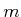be the number of polynomials in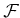, which are used to define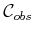. Recall from Section 4.3.3 how quickly this grows for simple examples. Let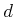be the maximum degree among the polynomials in. The maximum degree of the resulting polynomials is bounded by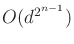, and the total number of polynomials is bounded by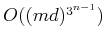. The total running time required to use cylindrical algebraic decomposition for motion planning is bounded by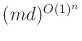.6.10 Note that the algorithm is doubly exponential in dimensionbut polynomial inand. It can theoretically be declared to be efficient on a space of motion planning problems of bounded dimension (although, it certainly is not efficient for motion planning in any practical sense).

Since the general problem is PSPACE-complete, it appears unavoidable that a complete, general motion planning algorithm will require a running time that is exponential in dimension. Since cylindrical algebraic decomposition is doubly exponential, it led many in the 1980s to wonder whether this upper bound could be lowered. This was achieved by Canny's roadmap algorithm, for which the running time is bounded by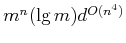. Hence, it is singly exponential, which appears very close to optimal because it is up against the lower bound that seems to be implied by PSPACE-hardness (and the fact that problems exist that require a roadmap with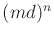connected components ). Much of the algorithm's complexity is due to finding a suitable deterministic perturbation to put the input polynomials into general position. A randomized algorithm can alternatively be used, for which the randomized expected running time is bounded by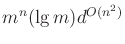. For a randomized algorithm , the randomized expected running time is still a worst-case upper bound, but averaged over random coin tosses'' that are introduced internally in the algorithm; it does not reflect any kind of average over the expected input distribution. Thus, these two bounds represent the best-known upper bounds for the general motion planning problem. Canny's algorithm may also be applied to solve the kinematic closure problems of Section 4.4, but the complexity does not reflect the fact that the dimension,, of the algebraic variety is less than, the dimension of. A roadmap algorithm that is particularly suited for this problem is introduced in , and its running time is bounded by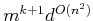. This serves as the best-known upper bound for the problems of Section 4.4.

Steven M LaValle 2020-08-14